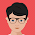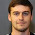# Javascript toString method with examples

### toString() method

toString() method exists in every object type of javascript. This is very useful for Converting primitive type to a String object in javascript.

In this tutorial, We are going to learn the Number, String and Boolean toString() method with examples.

#### Number toString() method

toString() method is defined number type in javascript. Which convert a string to a number.  This is used to Convert Number to String in javascript
Syntax
``````NumberObject.toString([radix])
``````
Parameter - Radix is an optional value ranging from 2 to 36 if any value supplied out of this range, It throws 'RangeError: toString() radix argument must be between 2 and 36'
2 represents base
8 represents Octal Numeric value
Return - Return numeric value of a string
Example
Following is an example of Number.toString method
``````var numberObject = 50;
console.log(numberObject.toString());
console.log(numberObject.toString(2));
console.log(numberObject.toString(8));
console.log(numberObject.toString(16));
console.log(numberObject.toString(54));
``````
output is
``````50
110010
62
32
Uncaught RangeError: toString() radix argument must be between 2 and 36
``````

#### String toString() method

This method is used to return the string value.
Syntax
``````StringObject.toString()
``````
Example
Following is an example of a String.toString() method

``````var stringObject = "test string";
console.log(stringObject.toString());
``````
output is
``````
test string
``````

#### Boolean toString() method

This method return string value of a Boolean object. This is used to Convert Boolean to String in javascript
Syntax
``````BooleanObject.toString()
``````
Example
``````var falseObject = new Boolean(false);
console.log(falseObject.toString());
var booleanObjFalse = new Boolean(0);
var booleanObjTrue1 = new Boolean(1);
var booleanObjTrue2 = new Boolean(14);
console.log(booleanObjFalse.toString());
console.log(booleanObjTrue1.toString());
console.log(booleanObjTrue2.toString());
``````
Output is
``````false
false
true
true
``````

#### Kiranbabu